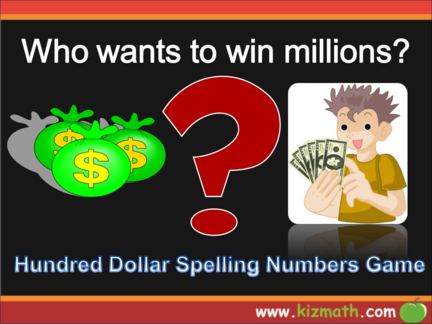# Spelling numbers - Jeopardy PowerPoint GameContributed by:1. Spell numbers from 1 to 10
2. Spell numbers from 10 to 20
3. Spell numbers from 20 to 30
4. Spell numbers from 30 to 40
5. Spell numbers from 40 to 50
1.
2. 1 to 10 10 to 20 20 to 30 30 to 40 40 to 50
\$100 \$100 \$100 \$100 \$100
\$200 \$200 \$200 \$200 \$200
\$300 \$300 \$300 \$300 \$300
\$400 \$400 \$400 \$400 \$400
3. How is this number spelt ?
4. How is this number spelt ?
5. How is this number spelt ?
6. How is this number spelt ?
7. How is this number spelt ?
8. How is this number spelt ?
9. How is this number spelt ?
10. How is this number spelt ?
11. How is this number spelt ?
12. How is this number spelt ?
13. How is this number spelt ?
14. How is this number spelt ?
15. How is this number spelt ?
16. How is this number spelt ?
17. How is this number spelt ?
18. How is this number spelt ?
19. How is this number spelt ?
20. How is this number spelt ?
21. How is this number spelt ?
22. How is this number spelt ?